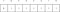# Two’s Complement : Binary Numbers for Programmers

## Signed Number Representation

Inside your machine you will want to represent numbers, that is to store numbers as binary somewhere. In order to store Integers within the computer there has to be a system. This is just one such system.

Difficulty: Beginner | Easy | Normal | Challenging

# Prerequisites:

• You should be familiar with Binary (guide HERE)

# Terminology

Binary: The name of the base 2 number system

Integer: A number that has no fractional part, that is no digits after the decimal point

One’s Complement: A value obtained by inverting the bits in a binary representation of a number

Radix: An alternative name for number base

Two’s Complement: The complement of a binary number with respect to 2^n

# Introduction

Your machine, whether it is a computer, mobile phone or games console needs to store `Integers` within the memory space that is avaliable. This article will explain how the system works.

## Example

Let us pretend we have a machine that has 8-bits of memory available.

Storing 1 in 8 bits of Two’s Complement

Using the usual `Binary` system we can store the `Denary` number 1 by making bit position 0 flipped to 1.

This is the same if we use `Two's Complement` or not.

Where `Two's Complement` is different is when tackling negative numbers.

Storing — 1 in 8 bits of Two’s Complement

We take 1 in `Binary` as usual, then follow a `Two's Complement` algorithm to convert the number.

We invert the digits (that is, 0 becomes 1 and 1 becomes 0)

so 11111111 represents -1 in `Two's Complement`

So we can put the three numbers together:

The maximum postitive number that can be stored in 8 bits of `Two's Complement` is 127The maximum number that can be stored in 8 bits of Two’s complement

Because the minimum value that can be stored is -128

Overflow in Two’s Complement

If you add 1 to 127 (stored in `Two's Complement` 8-bits)

you get to -127

If you wish to add -5 and 7 in `Two's Complement` it is unusually easy (which is one reason why `Two's Complement` is used.

There are 9-bits here! So we take off the first element. Actually this reveals the answer to be 2. Which luckily is correct!

# Two’s Complement Number wheel

## A Number Wheel for 4-bit numbers

For four bit numbers we could represent two’s complement through a rather attractive number wheel

representing positive and negative numbers as a wheel shows how the transition from positive to negative is handled — as well as how overflow is managed when a number too large for a number of bits is handled.

## One’s Complement

Similarly to `Two's complement` there is a `One's complement`. However, there is a problem in that there is a positive and a negative zero. This, and that in some arithmetic situations it does not behave quite as well as `Two's complement`.

# Conclusion:

`Two's complement` is the way we usually store numbers in a computer, as it makes it easy to complete arithmetic operations.

Now you know this, I hope it really helps you.

• Two’s Complement on Wikipedia (Link)

Any questions? You can get in touch with me here

Written by